# Let the python program automatically play Sudoku, and the second becomes the strongest brain!

2022-02-02 06:56:18The game interface is shown in the following figureOf course, there are many websites playing Sudoku , Now let's take the website as an example to demonstrate . I hope I can use Python Realize automatic calculation and fill in Sudoku game

Maybe the effect will be like the following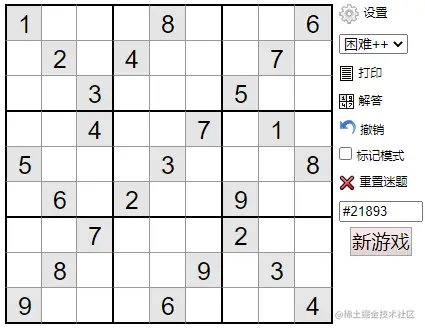I know the basic rules of Sudoku very well ：

1. Numbers 1-9 Only once in a row .
2. Numbers 1-9 It can only appear once in each column .
3. Numbers 1-9 Separated by thick solid lines in each 3x3 Only once in the palace .

How to let the program help us play this Sudoku game ？

Ideas ：

• We can go through web Automated test tool （ for example selenium） Open the page
• Parsing web pages to get table data
• Automatic table parsing in incoming handler
• Use the program to write the calculated Sudoku results automatically

Let's try to solve this problem step by step ：

## adopt Selenium Visit the target website

blog.csdn.net/as604049322…

First, through selenium Turn on the tour ：

``````from selenium import webdriver
browser = webdriver.Chrome()
Copy code ``````

If your selenium Has been installed correctly , Running the above code will open Google tour ：At this point, we can input... Directly in the controlled browser url visit , You can also use the code control browser to access the Sudoku game website ：

``````url = "https://www.sudoku.name/index.php?ln=cn&puzzle_num=&play=1&difficult=4&timer=&time_limit=0"
browser.get(url)
Copy code ``````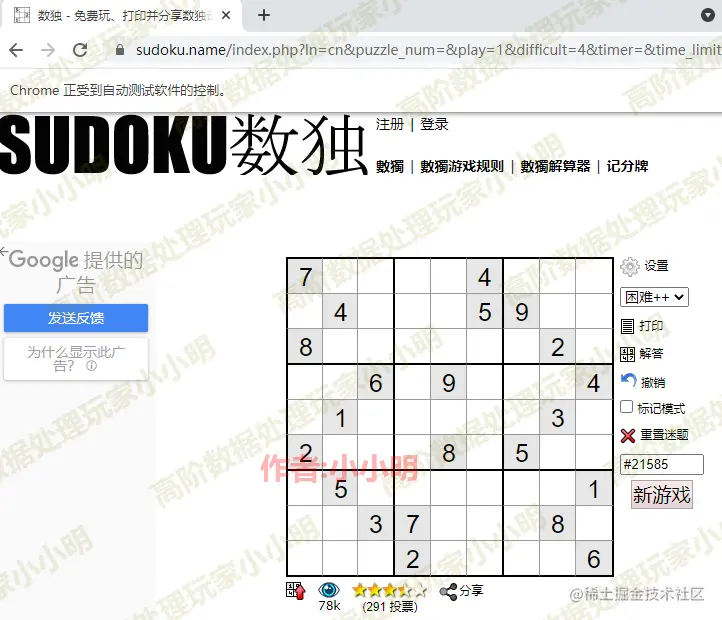inner PS： In the future, we still have to install a plug-in for Google browser to advertise

## Sudoku data extraction

Node analysis

table Node id by ：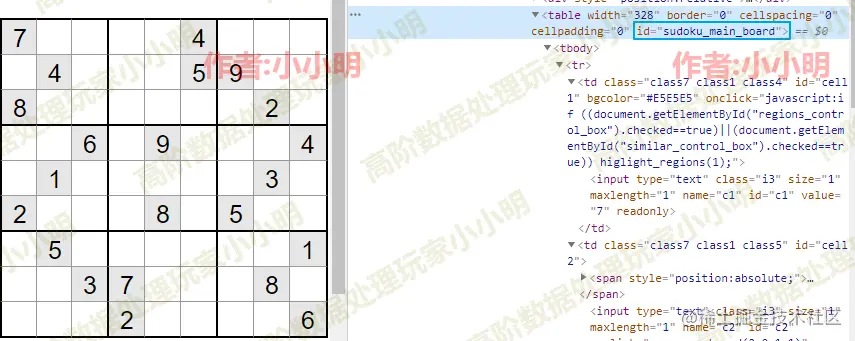Node values exist in value Properties of the ：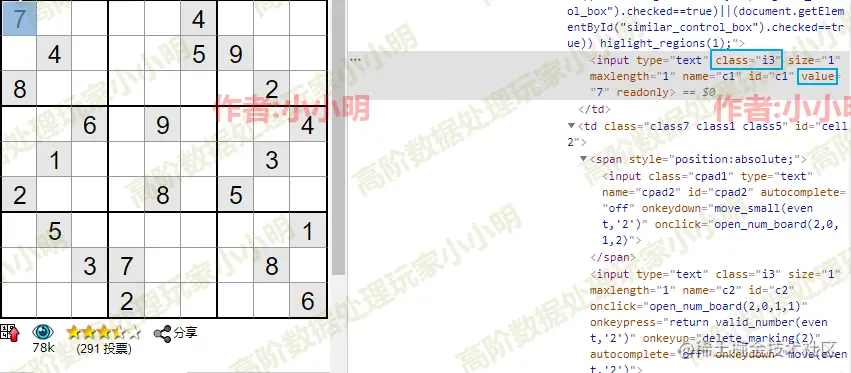Use Selenium That's the advantage of controlling the rover , You can let the program extract the data we need at any time .

First, get the goal table label ：

``````from selenium.webdriver.common.by import By
from selenium.webdriver.support.ui import WebDriverWait
from selenium.webdriver.support import expected_conditions as EC

wait = WebDriverWait(browser, 10)

table = wait.until(EC.element_to_be_clickable(
(By.CSS_SELECTOR, 'table#sudoku_main_board')))
Copy code ``````

Next, we extract the Sudoku data we need according to the analysis of the nodes ：

``````board = []
for tr in table.find_elements_by_xpath(".//tr"):
row = []
for input_e in tr.find_elements_by_xpath(".//input[@class='i3']"):
cell = input_e.get_attribute("value")
row.append(cell if cell else ".")
board.append(row)
board
Copy code ``````
``````[['7', '.', '.', '.', '.', '4', '.', '.', '.'],
['.', '4', '.', '.', '.', '5', '9', '.', '.'],
['8', '.', '.', '.', '.', '.', '.', '2', '.'],
['.', '.', '6', '.', '9', '.', '.', '.', '4'],
['.', '1', '.', '.', '.', '.', '.', '3', '.'],
['2', '.', '.', '.', '8', '.', '5', '.', '.'],
['.', '5', '.', '.', '.', '.', '.', '.', '1'],
['.', '.', '3', '7', '.', '.', '.', '8', '.'],
['.', '.', '.', '2', '.', '.', '.', '.', '6']]
Copy code ``````

Use... For all the positions that need to be filled in . Express .

## Sudoku program

How to use the above Sudoku program to calculate the result ？ This requires the thinking of logical algorithm .

The most basic way to solve this kind of problem is through recursion + The backtracking algorithm traverses all possible filling methods to verify the effectiveness one by one , Until there's no conflict . In the process of recursion , If the current blank can't fill in any number , So go back .

On this basis , We can use bit operations to optimize . In general, we need to use a length of 99 Indicates whether each number has ever appeared , By bit operation , Using only one integer can indicate whether each number has ever appeared . For example, binary tables (011000100) Representation number 3,7,8 It has appeared .

Specifically speaking, the final program is relatively complicated , Can not understand the logic of the code can be directly copied and pasted ：

``````def solveSudoku(board):
def flip(i: int, j: int, digit: int):
line[i] ^= (1 << digit)
column[j] ^= (1 << digit)
block[i // 3][j // 3] ^= (1 << digit)

def dfs(pos: int):
nonlocal valid
if pos == len(spaces):
valid = True
return

i, j = spaces[pos]
mask = ~(line[i] | column[j] | block[i // 3][j // 3]) & 0x1ff
digit = bin(digitMask).count("0") - 1
flip(i, j, digit)
board[i][j] = str(digit + 1)
dfs(pos + 1)
flip(i, j, digit)
if valid:
return

line =  * 9
column =  * 9
block = [ * 3 for _ in range(3)]
valid = False
spaces = list()

for i in range(9):
for j in range(9):
if board[i][j] == ".":
spaces.append((i, j))
else:
digit = int(board[i][j]) - 1
flip(i, j, digit)

dfs(0)
Copy code ``````

Then let's run ：

``````solveSudoku(board)
board
Copy code ``````
``````[['7', '2', '9', '3', '6', '4', '1', '5', '8'],
['3', '4', '1', '8', '2', '5', '9', '6', '7'],
['8', '6', '5', '9', '7', '1', '4', '2', '3'],
['5', '3', '6', '1', '9', '2', '8', '7', '4'],
['9', '1', '8', '5', '4', '7', '6', '3', '2'],
['2', '7', '4', '6', '8', '3', '5', '1', '9'],
['6', '5', '2', '4', '3', '8', '7', '9', '1'],
['4', '9', '3', '7', '1', '6', '2', '8', '5'],
['1', '8', '7', '2', '5', '9', '3', '4', '6']]
Copy code ``````

You can see , The program has calculated the result of Sudoku .

But for data ：

``````[['.', '.', '.', '6', '.', '.', '.', '3', '.'],
['5', '.', '.', '.', '.', '.', '6', '.', '.'],
['.', '9', '.', '.', '.', '5', '.', '.', '.'],
['.', '.', '4', '.', '1', '.', '.', '.', '6'],
['.', '.', '.', '4', '.', '3', '.', '.', '.'],
['8', '.', '.', '.', '9', '.', '5', '.', '.'],
['.', '.', '.', '7', '.', '.', '.', '4', '.'],
['.', '.', '5', '.', '.', '.', '.', '.', '8'],
['.', '3', '.', '.', '.', '8', '.', '.', '.']]
Copy code ``````

The time consumption of the above algorithm is up to 17 second , We need to continue to optimize the algorithm ：

``````def solveSudoku(board: list) -> None:
def flip(i: int, j: int, digit: int):
line[i] ^= (1 << digit)
column[j] ^= (1 << digit)
block[i // 3][j // 3] ^= (1 << digit)

def dfs(pos: int):
nonlocal valid
if pos == len(spaces):
valid = True
return

i, j = spaces[pos]
mask = ~(line[i] | column[j] | block[i // 3][j // 3]) & 0x1ff
digit = bin(digitMask).count("0") - 1
flip(i, j, digit)
board[i][j] = str(digit + 1)
dfs(pos + 1)
flip(i, j, digit)
if valid:
return

line =  * 9
column =  * 9
block = [ * 3 for _ in range(3)]
valid = False
spaces = list()

for i in range(9):
for j in range(9):
if board[i][j] != ".":
digit = int(board[i][j]) - 1
flip(i, j, digit)

while True:
modified = False
for i in range(9):
for j in range(9):
if board[i][j] == ".":
mask = ~(line[i] | column[j] |
block[i // 3][j // 3]) & 0x1ff
if not (mask & (mask - 1)):
digit = bin(mask).count("0") - 1
flip(i, j, digit)
board[i][j] = str(digit + 1)
modified = True
if not modified:
break

for i in range(9):
for j in range(9):
if board[i][j] == ".":
spaces.append((i, j))

dfs(0)
Copy code ``````

Run again ：

``````solveSudoku(board)
board
Copy code ``````

Time consuming only 3.2 second , A lot of performance improvements .

Optimization idea ： If a blank space has only one number to fill in , That is, its corresponding b Values and b-1 After bitwise sum operation, we get 0（ namely b Only one binary bit in is 1）. here , We can then determine the number of blanks to be filled in , Instead of waiting for recursion to deal with it .

What we need to do next is to put the result in the corresponding position , After all, it's hard to knock yourself .

## Write the results back to the web page

about Selenium, We can simulate a human click on a button and send a keyboard operation .

Now let's go through it again table label , And use click and send_keys Method ：

``````for i, tr in enumerate(table.find_elements_by_xpath(".//tr")):
for j, input_e in enumerate(tr.find_elements_by_xpath(".//input[@class='i3']")):
if input_e.get_attribute("readonly") == "true":
continue
input_e.click()
input_e.clear()
input_e.send_keys(board[i][j])
Copy code ``````

The effect in the process of operation ：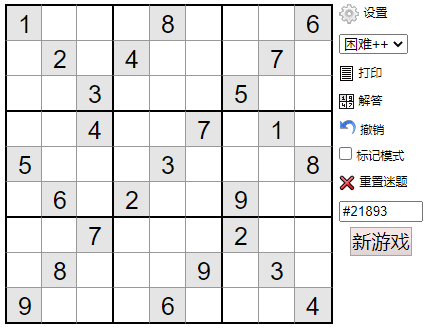Ashes level Sudoku player proves ：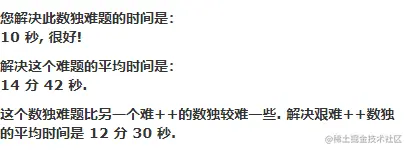others 14 minute , You use the program 10 Seconds to complete .

use Python I finally experienced it once “ Most brain ” I feel it. , Let me pretend to be B Go to

#### At the end of the article

Your favorite collection is my greatest encouragement ！ Welcome to follow me , Share Python dried food , communication Python technology . What's your opinion on the article , Or any technical problems , Welcome to leave a message and discuss in the comment area ！